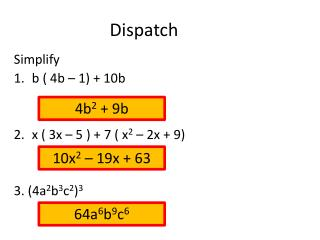DownloadDownload PresentationDispatch

# Dispatch

Télécharger la présentation## Dispatch

- - - - - - - - - - - - - - - - - - - - - - - - - - - E N D - - - - - - - - - - - - - - - - - - - - - - - - - - -
##### Presentation Transcript

1. Dispatch 4b2 + 9b 10x2 – 19x + 63 64a6b9c6 Simplify b ( 4b – 1) + 10b x ( 3x – 5 ) + 7 ( x2 – 2x + 9) 3. (4a2b3c2)3

2. Multiplying Polynomials Objective: We will be able to multiply polynomials using the Distributive Property, FOIL Method, and Magic Box Method. Standard: 10.0

3. Concept Task (x + 3)(x + 2) x2 + 5x + 6 (8d + 3)(5d + 2) 40d2 + 31d + 6

4. DISTRIBUTIVE PROPERTY GP (x + 3)(x + 2) Step 1: DP (x + 3)(x + 2) = x(x) + x(2) + 3(x) + 3(2) x2 + 2x + 3x + 6 Step 2: CLT x2 + 5x + 6

5. LETS REVIEW SOME VOCABULARY Coefficient (x + 3)(x + 2) x2 + 5x + 6 Linear Term Quadratic Term Constant Term

6. (4h – 2)(4h – 1) YT (2m + 2)(3m – 3) 6m2 – 6 (y – 2)(y + 8) y2 + 6y - 16 The length of a rectangle is 4h + 5and width h + 7. What is the area? 4h2 + 33h + 35

7. F: First Term O: Outer Term I: Inner Term L: Last Term FOIL METHOD GP (y + 4)(y – 3) (y + 4)(y – 3) F (y)(y) O (y)(-3) I (4)(y) L (4)(-3) y2 -3y + 4y - 12 y2 + y - 12

8. F: First Term O: Outer Term I: Inner Term L: Last Term FOIL METHOD GP (7x – 4)(5x – 1) (7x – 4)(5x – 1) F (7x)(5x) O (7x)(-1) I (-4)(5x) L (-4)(-1) 35x2 – 7x-20x + 4 35x2 -27x + 4

9. F: First Term O: Outer Term I: Inner Term L: Last Term YT (2w – 5)(w + 7) 2w2 + 9w – 35 (5m – 6)(5m – 6) 25m2– 60m+ 36

10. MAGIC BOX METHOD GP (p – 4)(p + 2) p – 4 X p +2 p2 -4p 2p -8 p2 – 2p – 8

11. MAGIC BOX METHOD GP (5a – 2)(2a– 3) 5a – 2 X 2a – 3 10a2 -4a – 15p 6 10a2 – 19p +6

12. YT (3c +1)(c – 2) (d – 1)(5d – 4) (4c + 1)(2c + 1)

13. MAGIC BOX METHOD GP (3c +1)(c – 2) 3c +1 X c – 2 3c2 c -6c -2 3c2 – 5c – 2

14. MAGIC BOX METHOD GP (d– 1)(5d – 4) d – 1 X 5d – 4 5d2 –5d -4d 4 5d2 – 9d+4

15. MAGIC BOX METHOD GP (4c + 1)(2c + 1) 4c + 1 X 2c +1 8c2 2c 4c 1 8c2+ 6c+1

16. Daily Practice Study Guide Intervention Worksheet Pg. 97 1-18 EVEN REMINDER: QUIZ TOMORROW PERIOD 1 1/18/13 REMINDER: TUTORING TODAY 3:50-4:50 LIBRARYGET EXTRA POINTS!!!

17. What if we have….. (p + 4)(p2 + 2p – 7) p( p2 + 2p – 7) + 4(p2 + 2p – 7) (8p) (-28) (-7p) (p3) + (2p2) (4p2) p3+ 6p2 + p – 28

18. F: First Term O: Outer Term I: Inner Term L: Last Term YT (2w – 5)(w + 7) 2w2 + 9w – 35 (5m – 6)(5m – 6) 25m2– 60m+ 36 The length of a rectangle is 10r – 4. The width is 10r + 4. What is the Area of the Rectangle? 100r2 – 16

19. A B C D A.Find (x + 2)(x – 3). A.x2 + x – 6 B.x2 – x – 6 C.x2 + x + 6 D.x2 + x + 5

20. A B C D B. Find (3x + 5)(2x – 6). A. 5x2 – 8x + 30 B. 6x2 + 28x – 1 C. 6x2 – 8x – 30 D. 6x – 30

21. Dispatch PA #3 Problems -5p3 – 9p2 + 5p Write an expression to represent the area of the rectangle 2x – 5 x + 4 Area= 2x2 + 3x – 20 Simplify Subtract (6p3 + 3p2 – 7p) from (p3 – 6p2 – 2p) 2.

22. LETS REVIEW F: First Term O: Outer Term I: Inner Term L: Last Term GP (c – 9)(c + 3) (c – 9)(c + 3) F (c)(c) O (c)(3) I (-9)(c) L (-9)(3) c2 +3c -9c-27 c2 – 6c – 27

23. YT (2x – 5)(3x2 – 4x + 1) 2x( 3x2 – 4x + 1) -5(3x2 – 4x + 1) 6x3– 23x2+ 22x – 5 (3k + 4)(7k2+ 2k– 9) 3k( 7k2 + 2k – 9) + 4(7k2 + 2k – 9) 21k3 – 34k2– 19k– 36

24. (n2 – 3n + 2 )(n2 + 5n – 4) YT n2(n2 + 5n – 4) – 3n(n2 + 5n – 4) + 2(n2+ 5n – 4) n4 + 2n3 – 17n2+ 22n – 8 (y2 + 7y – 1)(y2– 6y + 5) y2(y2 – 6y + 5) + 7y(y2 – 6y + 5) – 1(y2 – 6y + 5) y4+ y3– 38y2+ 41y – 5

25. Daily Practice Study Guide Intervention Worksheet Pg. 98 1-14

26. FOIL METHOD The length of a rectangle is 8d + 3. The width is 5d + 2. What is the Area of the Rectangle? GP F: First Term O: Outer Term I: Inner Term L: Last Term (8d + 3)(5d + 2) F (8d)(5d) O (8d)(2) I (3)(5d) L (3)(2) 40d2 + 16d +15d + 6 40d2 + 31d + 6